## 2. Layer Modes

GIMP has twenty-one layer modes. Layer modes are also sometimes called blending modes. Selecting a layer mode changes the appearance of the layer or image, based on the layer or layers beneath it. If there is only one layer, the layer mode has no effect. There must therefore be at least two layers in the image to be able to use layer modes.

You can set the layer mode in the Mode menu in the Layers dialog. GIMP uses the layer mode to determine how to combine each pixel in the top layer with the pixel in the same location in the layer below it.

BemærkThere is a drop-down list in the Toolbox options box which contains modes that affect the painting tools in a similar way to the layer modes. You can use all of the same modes for painting that are available for layers, and there are two additional modes just for the painting tools. See Afsnit 3.3, “Brush Tools (Pencil, Paintbrush, Airbrush)”.

Layer modes permit complex color changes in the image. They are often used with a new layer which acts as a kind of mask. For example, if you put a solid white layer over an image and set the layer mode of the new layer to Saturation, the underlying visible layers will appear in shades of gray.

Figur 8.8. Images (masks) for layer mode examples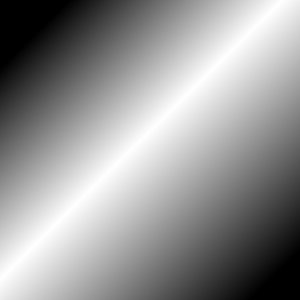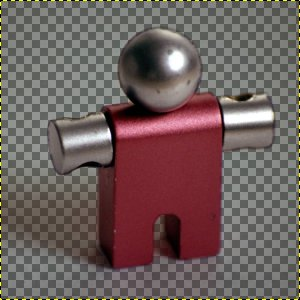Figur 8.9. Images (backgrounds) for layer mode examples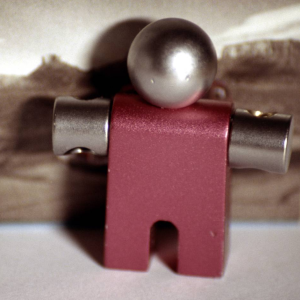Key fobDucks

In the descriptions of the layer modes below, the equations are also shown. This is for those who are curious about the mathematics of the layer modes. You do not need to understand the equations in order to use the layer modes effectively, however.

The equations are in a shorthand notation. For example, the equation

Ligning 8.1. Example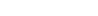means, For each pixel in the upper (Mask)and lower (Image) layer, add each of the corresponding color components together to form the E resulting pixel's color. Pixel color components must always be between 0 and 255.

BemærkUnless the description below says otherwise, a negative color component is set to 0 and a color component larger than 255 is set to 255.

The examples below show the effects of each of the modes.

Since the results of each mode vary greatly depending upon the colors on the layers, these images can only give you a general idea of how the modes work. You are encouraged to try them out yourself. You might start with two similar layers, where one is a copy of the other, but slightly modified (by being blurred, moved, rotated, scaled, color-inverted, etc.) and seeing what happens with the layer modes.

Normal

Figur 8.10. Example for layer mode Normal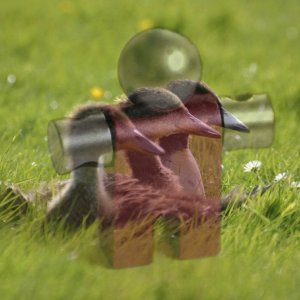Both images are blended into each other with the same intensity.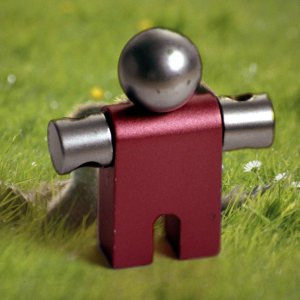With 100% opacity only the upper layer is shown when blending with Normal.

Normal mode is the default layer mode. The layer on top covers the layers below it. If you want to see anything below the top layer when you use this mode, the layer must have some transparent areas.

The equation is:

Ligning 8.2. Equation for layer mode Normal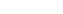Opløs

Figur 8.11. Example for layer mode Dissolve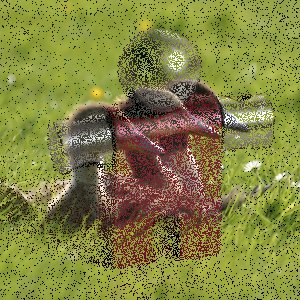Both images are blended into each other with the same intensity.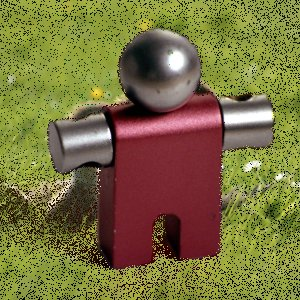With 100% opacity only the upper layer is shown when blending with dissolve.

Dissolve mode dissolves the upper layer into the layer beneath it by drawing a random pattern of pixels in areas of partial transparency. It is useful as a layer mode, but it is also often useful as a painting mode.

This is especially visible along the edges within an image. It is easiest to see in an enlarged screenshot. The image on the left illustrates Normal layer mode (enlarged) and the image on the right shows the same two layers in Dissolve mode, where it can be clearly seen how the pixels are dispersed.

Figur 8.12. Enlarged screenshots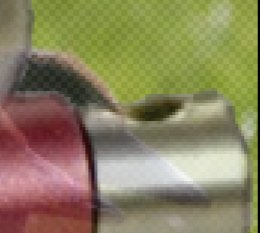Normal mode.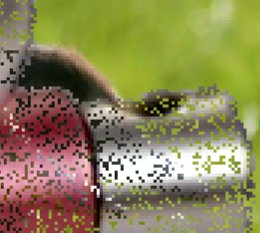Dissolve mode.

Gang

Figur 8.13. Example for layer mode Multiply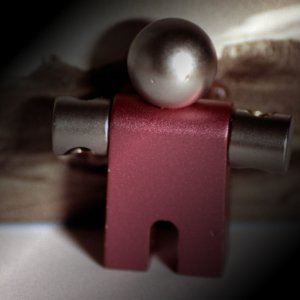Mask 1 is used as upper layer with 100% opacity.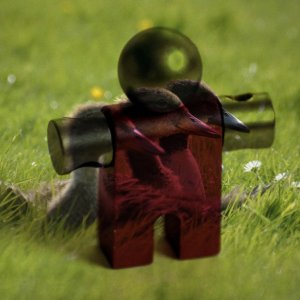Mask 2 is used as upper layer with 100% opacity.

Multiply mode multiplies the pixel values of the upper layer with those of the layer below it and then divides the result by 255. The result is usually a darker image. If either layer is white, the resulting image is the same as the other layer (1 * I = I). If either layer is black, the resulting image is completely black (0 * I = 0).

The equation is:

Ligning 8.3. Equation for layer mode Multiply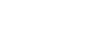The mode is commutative; the order of the two layers doesn't matter.

Divide

Figur 8.14. Example for layer mode Divide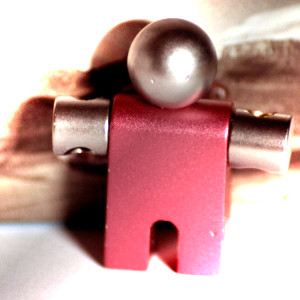Mask 1 is used as upper layer with 100% opacity.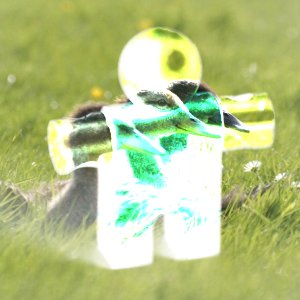Mask 2 is used as upper layer with 100% opacity.

Divide mode multiplies each pixel value in the lower layer by 256 and then divides that by the corresponding pixel value of the upper layer plus one. (Adding one to the denominator avoids dividing by zero.) The resulting image is often lighter, and sometimes looks burned out.

The equation is:

Ligning 8.4. Equation for layer mode Divide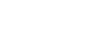Skærm

Figur 8.15. Example for layer mode ScreenMask 1 is used as upper layer with 100% opacity.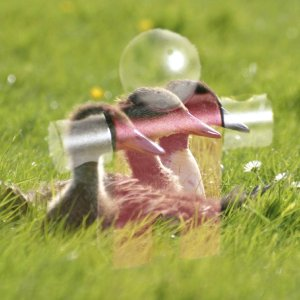Mask 2 is used as upper layer with 100% opacity.

Screen mode inverts the values of each of the visible pixels in the two layers of the image. (That is, it subtracts each of them from 255.) Then it multiplies them together, divides by 255 and inverts this value again. The resulting image is usually brighter, and sometimes washed out in appearance. The exceptions to this are a black layer, which does not change the other layer, and a white layer, which results in a white image. Darker colors in the image appear to be more transparent.

The equation is:

Ligning 8.5. Equation for layer mode Screen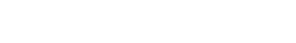The mode is commutative; the order of the two layers doesn't matter.

Læg over

Figur 8.16. Example for layer mode Overlay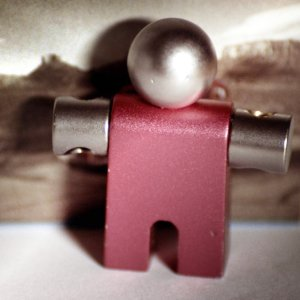Mask 1 is used as upper layer with 100% opacity.Mask 2 is used as upper layer with 100% opacity.

Overlay mode inverts the pixel value of the lower layer, multiplies it by two times the pixel value of the upper layer, adds that to the original pixel value of the lower layer, divides by 255, and then multiplies by the pixel value of the original lower layer and divides by 255 again. It darkens the image, but not as much as with Multiply mode.

The equation is: 

Ligning 8.6. Equation for layer mode Overlay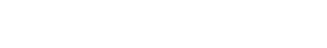Dodge

Figur 8.17. Example for layer mode Dodge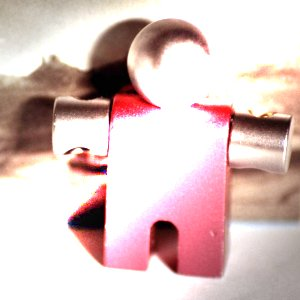Mask 1 is used as upper layer with 100% opacity.Mask 2 is used as upper layer with 100% opacity.

Dodge mode multiplies the pixel value of the lower layer by 256, then divides that by the inverse of the pixel value of the top layer. The resulting image is usually lighter, but some colors may be inverted.

In photography, dodging is a technique used in a darkroom to decrease the exposure in particular areas of the image. This brings out details in the shadows. When used for this purpose, dodge may work best on Grayscale images and with a painting tool, rather than as a layer mode.

The equation is:

Ligning 8.7. Equation for layer mode Dodge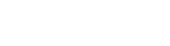Burn

Figur 8.18. Example for layer mode Burn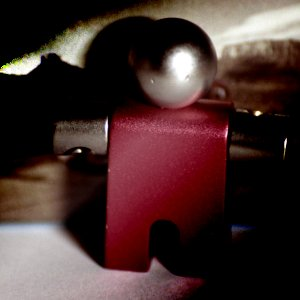Mask 1 is used as upper layer with 100% opacity.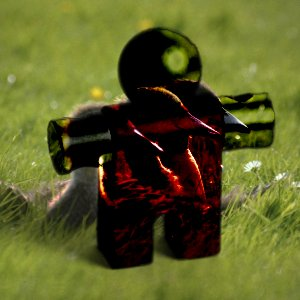Mask 2 is used as upper layer with 100% opacity.

Burn mode inverts the pixel value of the lower layer, multiplies it by 256, divides that by one plus the pixel value of the upper layer, then inverts the result. It tends to make the image darker, somewhat similar to Multiply mode.

In photography, burning is a technique used in a darkroom to increase the exposure in particular areas of the image. This brings out details in the highlights. When used for this purpose, burn may work best on Grayscale images and with a painting tool, rather than as a layer mode.

The equation is:

Ligning 8.8. Equation for layer mode Burn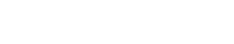Hard light

Figur 8.19. Example for layer mode Hard light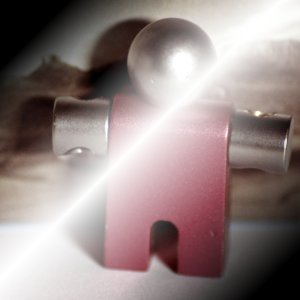Mask 1 is used as upper layer with 100% opacity.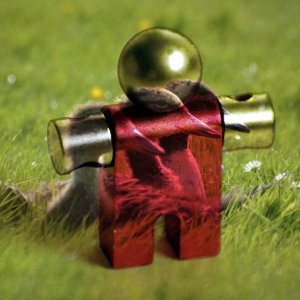Mask 2 is used as upper layer with 100% opacity.

Hard light mode is rather complicated because the equation consists of two parts, one for darker colors and one for brighter colors. If the pixel color of the upper layer is greater than 128, the layers are combined according to the first formula shown below. Otherwise, the pixel values of the upper and lower layers are multiplied together and multiplied by two, then divided by 256. You might use this mode to combine two photographs and obtain bright colors and sharp edges.

The equation is complex and different according to the value >128 or ≤ 128:

Ligning 8.9. Equation for layer mode Hard light, M > 128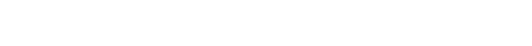Ligning 8.10. Equation for layer mode Hard light, M ≤ 128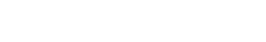Soft light

Figur 8.20. Example for layer mode Soft light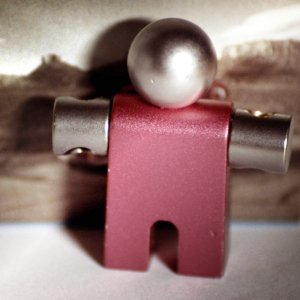Mask 1 is used as upper layer with 100% opacity.Mask 2 is used as upper layer with 100% opacity.

Soft light is not related to Hard light in anything but the name, but it does tend to make the edges softer and the colors not so bright. It is similar to Overlay mode. In some versions of GIMP, Overlay mode and Soft light mode are identical.

The equation is complicated. It needs Rs, the result of Screen mode :

Ligning 8.11. Equation for layer mode Screen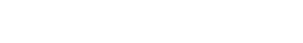Ligning 8.12. Equation for layer mode Soft light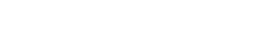Grain extract

Figur 8.21. Example for layer mode Grain extract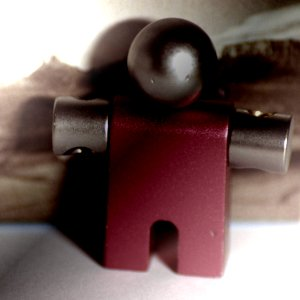Mask 1 is used as upper layer with 100% opacity.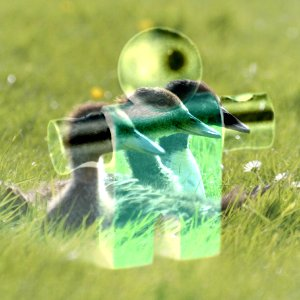Mask 2 is used as upper layer with 100% opacity.

Grain extract mode is supposed to extract the film grain from a layer to produce a new layer that is pure grain, but it can also be useful for giving images an embossed appearance. It subtracts the pixel value of the upper layer from that of the lower layer and adds 128.

The equation is:

Ligning 8.13. Equation for layer mode Grain extract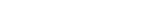Grain merge

There are two more layer modes, but these are available only for painting tools. See Painting Modes for detailed information.

Figur 8.22. Example for layer mode Grain merge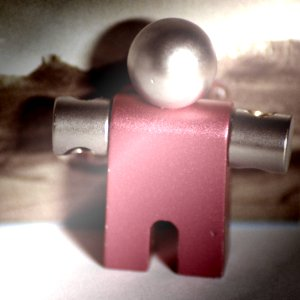Mask 1 is used as upper layer with 100% opacity.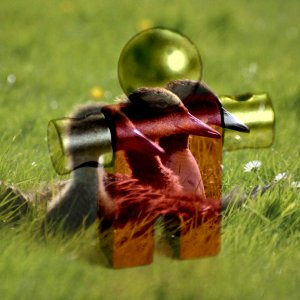Mask 2 is used as upper layer with 100% opacity.

Grain merge mode merges a grain layer (possibly one created from the Grain extract mode) into the current layer, leaving a grainy version of the original layer. It does just the opposite of Grain extract. It adds the pixel values of the upper and lower layers together and subtracts 128.

The equation is:

Ligning 8.14. Equation for layer mode Grain merge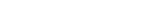Difference

Figur 8.23. Example for layer mode Difference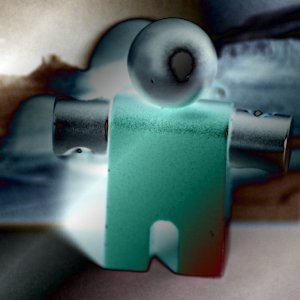Mask 1 is used as upper layer with 100% opacity.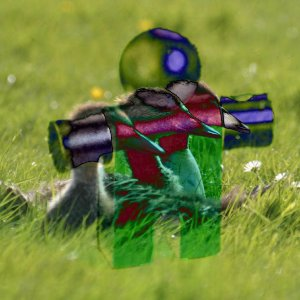Mask 2 is used as upper layer with 100% opacity.

Difference mode subtracts the pixel value of the upper layer from that of the lower layer and then takes the absolute value of the result. No matter what the original two layers look like, the result looks rather odd. You can use it to invert elements of an image.

The equation is:

Ligning 8.15. Equation for layer mode Difference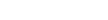The mode is commutative; the order of the two layers doesn't matter.

Læg til

Figur 8.24. Example for layer mode Addition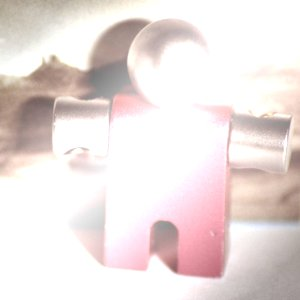Mask 1 is used as upper layer with 100% opacity.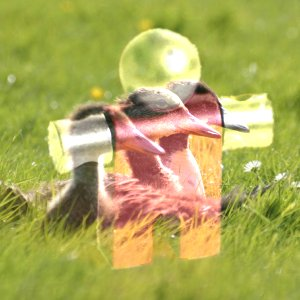Mask 2 is used as upper layer with 100% opacity.

Addition mode is very simple. The pixel values of the upper and lower layers are added to each other. The resulting image is usually lighter. The equation can result in color values greater than 255, so some of the light colors may be set to the maximum value of 255.

The equation is:

Ligning 8.16. Equation for layer mode Addition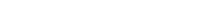The mode is commutative; the order of the two layers doesn't matter.

Subtrahér

Figur 8.25. Example for layer mode Subtract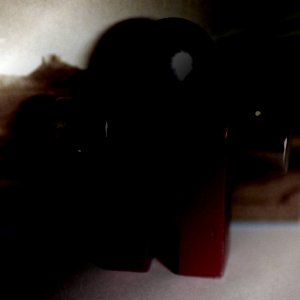Mask 1 is used as upper layer with 100% opacity.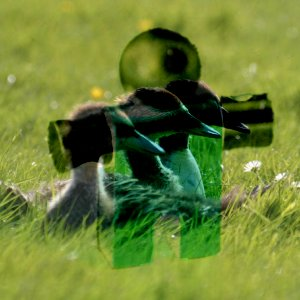Mask 2 is used as upper layer with 100% opacity.

Subtract mode subtracts the pixel values of the upper layer from the pixel values of the lower layer. The resulting image is normally darker. You might get a lot of black or near-black in the resulting image. The equation can result in negative color values, so some of the dark colors may be set to the minimum value of 0.

The equation is:

Ligning 8.17. Equation for layer mode Subtraction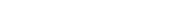Formørk kun

Figur 8.26. Example for layer mode Darken only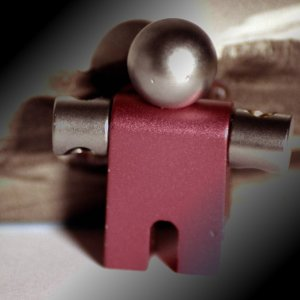Mask 1 is used as upper layer with 100% opacity.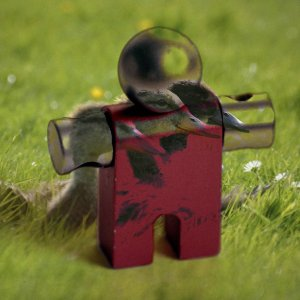Mask 2 is used as upper layer with 100% opacity.

Darken only mode compares each component of each pixel in the upper layer with the corresponding one in the lower layer and uses the smaller value in the resulting image. Completely white layers have no effect on the final image and completely black layers result in a black image.

The equation is:

Ligning 8.18. Equation for layer mode Darken only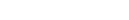The mode is commutative; the order of the two layers doesn't matter.

Lighten only

Figur 8.27. Example for layer mode Lighten only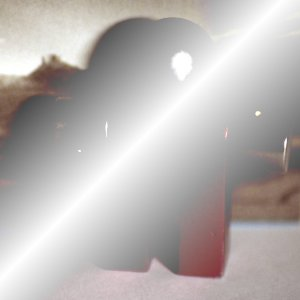Mask 1 is used as upper layer with 100% opacity.Mask 2 is used as upper layer with 100% opacity.

Lighten only mode compares each component of each pixel in the upper layer with the corresponding one in the lower layer and uses the larger value in the resulting image. Completely black layers have no effect on the final image and completely white layers result in a white image.

The equation is:

Ligning 8.19. Equation for layer mode Lighten only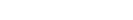The mode is commutative; the order of the two layers doesn't matter.

Farvetone

Figur 8.28. Example for layer mode Hue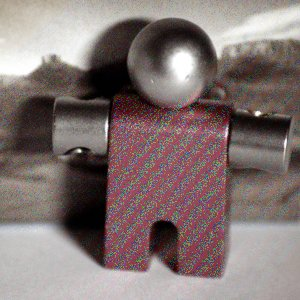Mask 1 is used as upper layer with 100% opacity.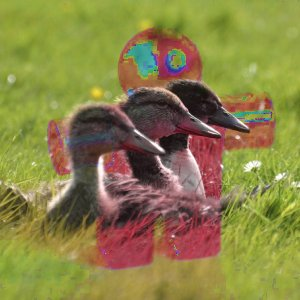Mask 2 is used as upper layer with 100% opacity.

Hue mode uses the hue of the upper layer and the saturation and value of the lower layer to form the resulting image. However, if the saturation of the upper layer is zero, the hue is taken from the lower layer, too.

Mætning

Figur 8.29. Example for layer mode Saturation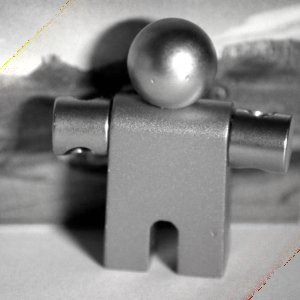Mask 1 is used as upper layer with 100% opacity.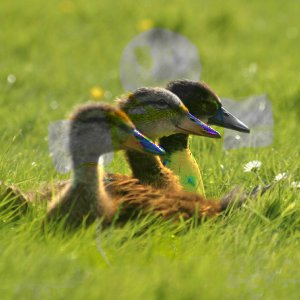Mask 2 is used as upper layer with 100% opacity.

Saturation mode uses the saturation of the upper layer and the hue and value of the lower layer to form the resulting image.

Farve

Figur 8.30. Example for layer mode Color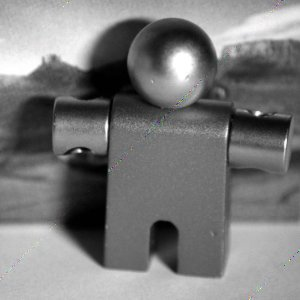Mask 1 is used as upper layer with 100% opacity.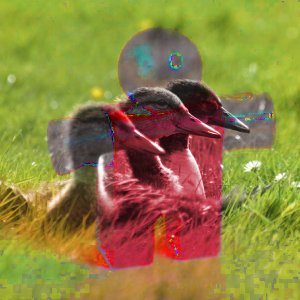Mask 2 is used as upper layer with 100% opacity.

Color mode uses the hue and saturation of the upper layer and the value of the lower layer to form the resulting image.

Værdi

Figur 8.31. Example for layer mode Value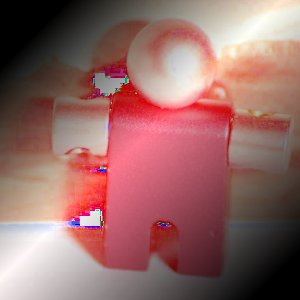Mask 1 is used as upper layer with 100% opacity.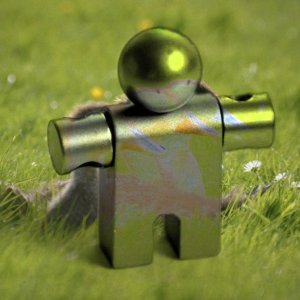Mask 2 is used as upper layer with 100% opacity.

Value mode uses the value of the upper layer and the saturation and hue of the lower layer to form the resulting image. You can use this mode to reveal details in dark and light areas of an image without changing the saturation.

Each layer in an image can have a different layer mode. (Of course, the layer mode of the bottom layer of an image has no effect.) The effects of these layer modes are cumulative. The image shown below has three layers. The top layer consists of Wilber surrounded by transparency and has a layer mode of Difference. The second layer is solid light blue and has a layer mode of Addition. The bottom layer is filled with the Red Cubes pattern.

Figur 8.32. Multi layer example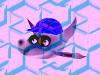GIMP also has similar modes which are used for the painting tools. These are the same twenty-one modes as the layer modes, plus additionally two modes which are specific to the painting tools. You can set these modes from the Mode menu in the Tools option dialog. In the equations shown above, the layer you are painting on is the lower layer and the pixels painted by the tool are the upper layer. Naturally, you do not need more than one layer in the image to use these modes, since they only operate on the current layer and the selected painting tool.

See Afsnit 3.1.3, “Paint Mode Examples” for a description of the two additional painting modes.

 The equation is the *theoretical* equation. Due to Bug #162395 , the actual equation is equivalent to Soft light. It is difficult to fix this bug without changing the appearance of existing images.